# PythonPractice

## exec vs setattr (time)

```import time

class A:
def __init__(self):
self.value = 1
self.vdict = {}
self.vdict['0'] = str("self.value")

start = time.time()
for i in range(1000):
setattr(self, self.vdict['0'], 5)
print('TIME(ms)  is=', 1000 * (time.time() - start))

start = time.time()
for i in range(1000):
exec(self.vdict['0'])
print('TIME(ms)  is=', 1000 * (time.time() - start))

if __name__ == '__main__':
classA = A()
```

## 进程与线程

### 信号回调函数只能在主线程处理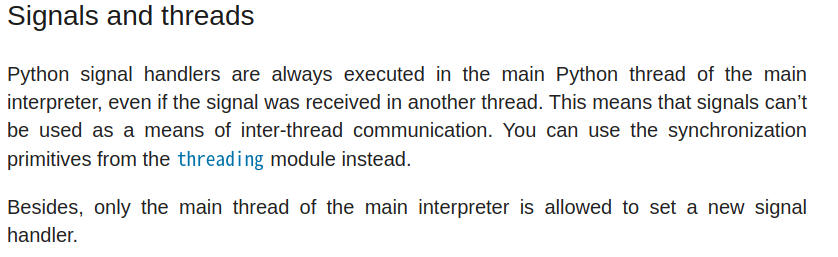python的 `信号回调函数` 的定义和执行只能在主线程中。若有主线程main和子线程A，则即使子线程收到了信号，也只会在主线程中执行。凡此，python的信号机制不能用于线程间通信。

### 自定义信号中断函数

```import signal
def keyboard_interrupt_handler(signal, frame):
pass
signal.signal(signal.SIGINT, keyboard_interrupt_handler)
```

### 信号的默认处理机制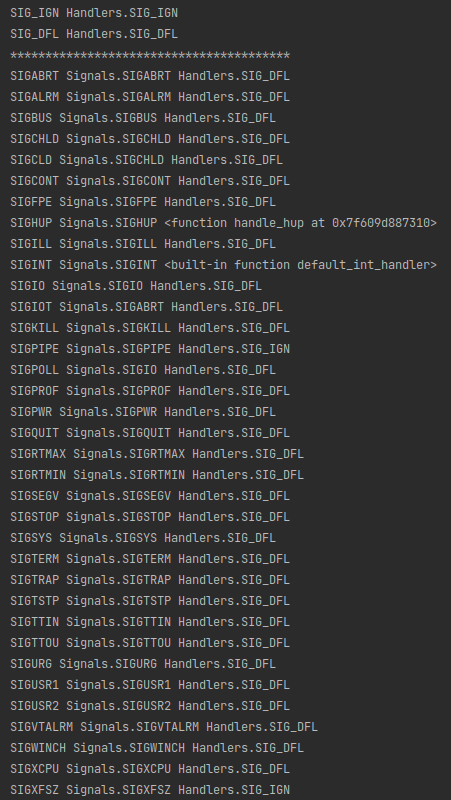### 锁

```from threading import Lock

lock = Lock()

# 线程1：
with lock:
# todo fun(variableA)

# 线程2：
with lock:
# todo fun(variableB)
```
• 需要访问同一资源的线程都需要上锁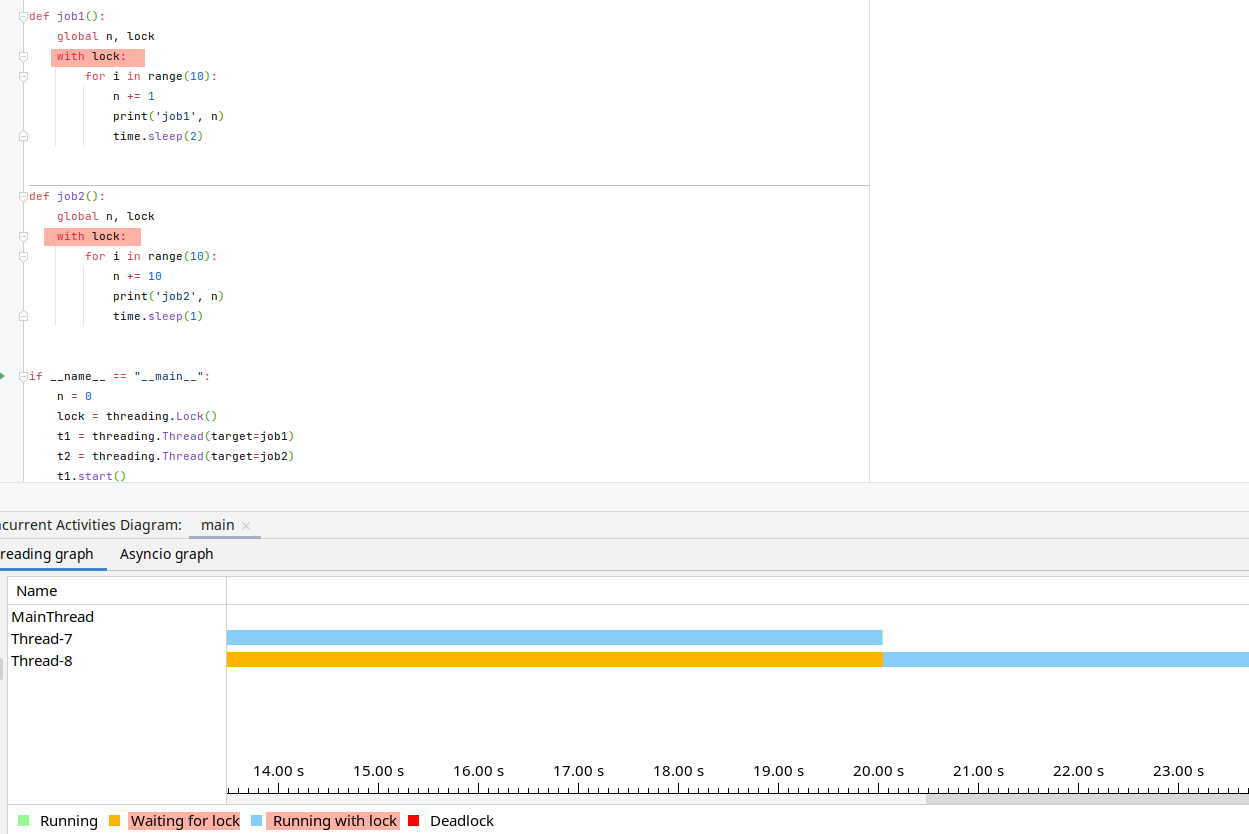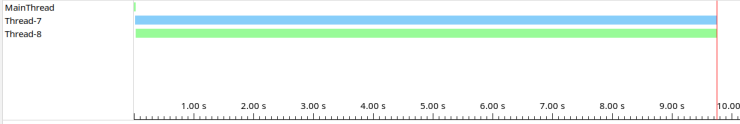## 构建配置文件

• 在大型深度学习模型中，通常需要使用配置文件存储参数

• 方法一：基于python构建，内部存储字典数据，然后到时import python文件（参考livox_detection）

```# config/config.py
CLASSES = ['car', 'bus', 'truck', 'pedestrian', 'bimo']
```

```# 另一文件用于调用该配置
import config.config as cfg
```
• 方法二：基于yaml存放配置参数，然后调用内置库读取（参考OpenPCDet）

```DATASET: 'KittiDataset'
DATA_PATH: '../data/kitti'
```

## python中的路径问题

```# e.g.
```

## 字符串处理

### 常见操作

```# 默认去开头/结尾的空格和换行符 \n
<str>.strip()
# 以某个符号为分界点进行分割（不包含该符号）；默认分割符为空格和换行符
<str>.split()
```

### 格式化处理

%s

%10s

%.2s

%10.2s

10位字符串，只保留两位的字符串

r"str"

u"str"

```num = 100
print(f"{num:0>4d}") # 0100
```

### 实例

• 读取文件P2字段的标定数据

```# 去头去尾（去\n等回车符）-> 以空格为分隔符进行分割 -> 去除'P2:'字符串 -> 类型转换(str->num) -> reshape 得到变换矩阵
np.array(lines.strip().split(' ')[1:], dtype=np.float32).reshape(3, 4)
```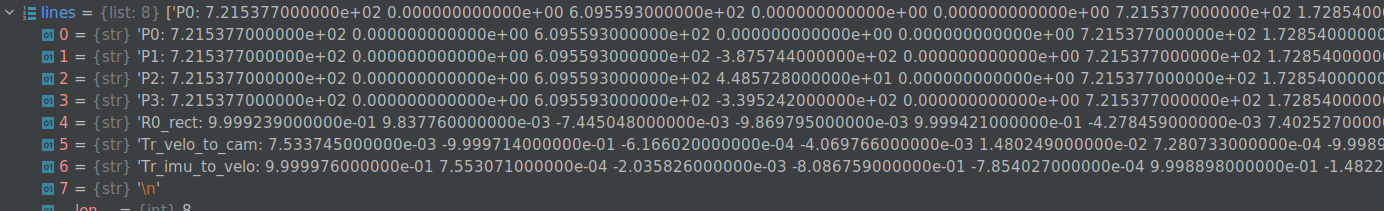## 深拷贝和浅拷贝

```# 浅拷贝：外层对象地址改变，内层对象地址不改变
copy.copy()
# 深拷贝：内外层对象地址均改变
copy.deepcopy()
```

## 语法

### 内置变量

#### 字典

```A = {"a": 1, "b": 2}
B = {"a": 3, "b": 3, "c": 4}
A.update(B)
print(A) # {'a': 3, 'b': 3, 'c': 4}
```

#### 列表

```# 列表翻转
A = [1,4,5,7]
A_rev = list(reversed(A)) # list(迭代器)

# 列表移除某个值（只能移除第一个出现的值）
A.remove(<list>)

A.append(B) # 将列表B以元素的形式加入到列表A中
A.extend(B) # 列表B的元素加入到列表B中
```

```# 交集
A & B
```

### 匿名函数

```add = lambda x,y:x + y
```

### 继承

• 子类不重写init方法，则实例化时自动调用父类的init方法；子类重写init方法后，又想调用父类的init则需要

```# 等价于：父类名称.__init__(self, 参数1, 参数2，...)
super(子类，self).__init__(参数1, 参数2, ...)
```
• 多继承时，如果子类没有重写init，实例时自动调用的是第一个类的init方法

## 实战

### 判断一个数（浮点数）是否是整数

```# 方法一：
(num).is_integer

# 方法二：
num % 1 == 0
```

### 编码转换

#### str和byte的转换

```# byte -> str
<byte>.decode()
# str -> byte
<str>.encode()
```

#### img和base64的转换

```# img转为base64
with open("img_path", 'rb') as f:
# 将图片编码成base64 string数据，
```

### 指定脚本运行的解释器

```
span.prompt1:before {
content: "\$ ";
}
span.prompt2:before {
content: "# ";
}

/usr/bin/env python

/usr/bin/python2.7

```